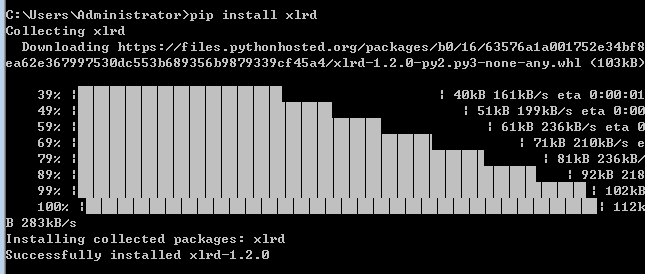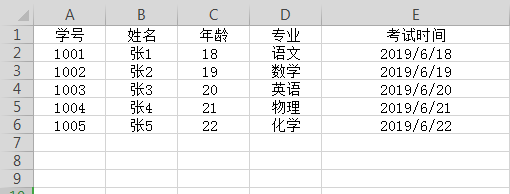# Python xlrd模块读取Excel表中的数据

1、xlrd库的安装

pip install xlrd2、xlrd模块的一些常用命令
①打开excel文件并创建对象存储

data = xlrd.open_workbook(文件路径)


②获取文件中所有工作表的名称

data.sheet_names()


③根据工作表的名称获取里面的行列内容

table = data.sheet_by_name('Sheet1')


④获取工作表的名称、行数、列数

name = table.name
rowNum = table.nrows
colNum = table.ncols


⑤获取单元格内容的3种方式

table.cell(i,j).value

table.cell_value(i,j)

table.row(i)[j].value


⑥获取单元格数据类型

table.cell(i,j).ctype

type(table.cell_value(i, j)


xlrd的数据类型有：

0 empty,
1 string,
2 number,
3 date,
4 boolean,
5 error

⑦获取工作表第一行的所有字段列表

table.row_values(0)


3、写一个自动获取excel表内容的类

import xlrd
from xlrd import xldate_as_tuple
import datetime
'''
xlrd中单元格的数据类型

0 empty,1 string, 2 number, 3 date, 4 boolean, 5 error
'''
class ExcelData():
# 初始化方法
def __init__(self, data_path, sheetname):
#定义一个属性接收文件路径
self.data_path = data_path
# 定义一个属性接收工作表名称
self.sheetname = sheetname
# 使用xlrd模块打开excel表读取数据
self.data = xlrd.open_workbook(self.data_path)
# 根据工作表的名称获取工作表中的内容（方式①）
self.table = self.data.sheet_by_name(self.sheetname)
# 根据工作表的索引获取工作表的内容（方式②）
# self.table = self.data.sheet_by_name(0)
# 获取第一行所有内容,如果括号中1就是第二行，这点跟列表索引类似
self.keys = self.table.row_values(0)
# 获取工作表的有效行数
self.rowNum = self.table.nrows
# 获取工作表的有效列数
self.colNum = self.table.ncols

# 定义一个读取excel表的方法
# 定义一个空列表
datas = []
for i in range(1, self.rowNum):
# 定义一个空字典
sheet_data = {}
for j in range(self.colNum):
# 获取单元格数据类型
c_type = self.table.cell(i,j).ctype
# 获取单元格数据
c_cell = self.table.cell_value(i, j)
if c_type == 2 and c_cell % 1 == 0:  # 如果是整形
c_cell = int(c_cell)
elif c_type == 3:
# 转成datetime对象
date = datetime.datetime(*xldate_as_tuple(c_cell,0))
c_cell = date.strftime('%Y/%d/%m %H:%M:%S')
elif c_type == 4:
c_cell = True if c_cell == 1 else False
sheet_data[self.keys[j]] = c_cell
# 循环每一个有效的单元格，将字段与值对应存储到字典中
# 字典的key就是excel表中每列第一行的字段
# sheet_data[self.keys[j]] = self.table.row_values(i)[j]
# 再将字典追加到列表中
datas.append(sheet_data)
# 返回从excel中获取到的数据：以列表存字典的形式返回
return datas
if __name__ == "__main__":
data_path = "ttt.xlsx"
sheetname = "Sheet1"
get_data = ExcelData(data_path, sheetname)
print(datas)[
{'学号': 1001, '姓名': '张1', '年龄': 18, '专业': '语文', '考试时间': '2019/18/06 09:00:00'},
{'学号': 1002, '姓名': '张2', '年龄': 19, '专业': '数学', '考试时间': '2019/19/06 09:00:00'},
{'学号': 1003, '姓名': '张3', '年龄': 20, '专业': '英语', '考试时间': '2019/20/06 09:00:00'},
{'学号': 1004, '姓名': '张4', '年龄': 21, '专业': '物理', '考试时间': '2019/21/06 09:00:00'},
{'学号': 1005, '姓名': '张5', '年龄': 22, '专业': '化学', '考试时间': '2019/22/06 09:00:00'}
]

posted @ 2019-06-21 21:12  Python及时行乐  阅读(70989)  评论(1编辑  收藏  举报